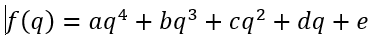# (help) Disciplined convex programming error: Invalid quadratic form(s): not a square

Hello. I find the optimal value using MATLAB and CVX.

But, when i run the code, it has some errors as follows:

다음 사용 중 오류가 발생함: times>times_q (line 84)
Disciplined convex programming error:
Invalid quadratic form(s): not a square.

오류 발생: cvx_binary_op (line 107)

오류 발생: .* (line 53)

오류 발생: * (line 35)

오류 발생: compute_Rq (line 22)
minimize( asquare(square(q)) + bqsquare(q) + csquare(q) + d*q + e )

오류 발생: Test (line 50)
Rq(i,j) = compute_Rq(i,j,Q,F,P);.

here is my code.

``````cvx_begin
variable q
minimize( a*square(square(q)) + b*q*square(q) + c*square(q) + d*q + e )
cvx_end
``````

where a,b,c,d,e is the real scalar number, and q is the scalar variable.
and my cost function is as follows:is there some fault? i cannot find why the error occurs.

somebody help me.

The problem as written is non-convex. However, if `a, b, c` are all nonnegative, and `x` is constrained to be nonnegative, then the program can be rewritten as:
``````cvx_begin
`pow_pos` constrains `x` to be nonnegative. Alternatively, `pow_p` could be used, which would do the same thing in this case.